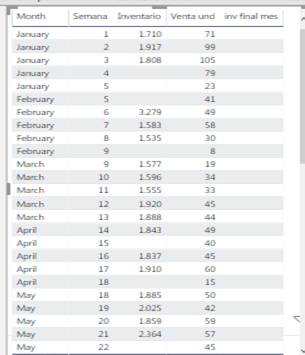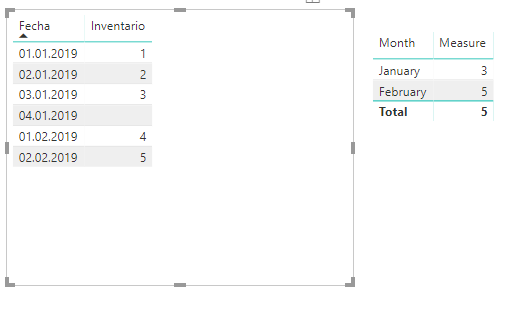cancel
Showing results for
Did you mean:Anonymous
Not applicable

## Extract last data from a month

Hello everyone,

I have this table and i just want to extract the last inventory of each month, i've been trying without succesfull results. I'd really appreciate some help:The expected result is as follows:Thank you

9 REPLIES 9Community Champion

Hi @Anonymous

try create a new table

``NewTable = summarize(Table;Table[Data].[Month];"lastinventory";LASTNONBLANK(Table[Inventario];1))``

or

``NewTable = summarize(Table;Table[Data].[MonthNo];"lastinventory";LASTNONBLANK(Table[Inventario];1))``

do not hesitate to give a kudo to useful posts and mark solutions as solution

do not hesitate to give a kudo to useful posts and mark solutions as solutionAnonymous
Not applicable

Hi @az38 ,

Thank you for your response, however, i didn't find the expected result.

Table = SUMMARIZE('total base','total base'[Fecha].[Month],"lastinv",LASTNONBLANK('total base'[Inventario],1))
The right one is the result of the table:
For example In January i just need 143947 as result.Thank you again.Community Champion

Hi @Anonymous

for january result is correct as I see

the right table says that you have a data from other monthes.

if you want to see onle january try

``Table = SUMMARIZE(FILTER('total base', MONTH('total_base'[[Fecha]])=1),'total base'[Fecha].[Month],"lastinv",LASTNONBLANK('total base'[Inventario],1))``

do not hesitate to give a kudo to useful posts and mark solutions as solution

do not hesitate to give a kudo to useful posts and mark solutions as solutionAnonymous
Not applicable

I have a data base with weekly sales and inventory. I can accumulate the sales during the year, but the inventory is the current state of stock in each week ( so the inventory of the month is the inventory of the last week of the month with data ).

I need a measure ( i suppose), that gives me the last week of inventory when i use each month in a visualization.

Thank you.Community Champion

So, @Anonymous the solution with summarized table doesnt satisfy you?

try a measure like

``Measure = calculate(lastnonblank('total base'[Inventario],1). allexcept('total base','total base'[Fecha]))``

then in visual leave onle month-member of 'total base'[Fecha] hierarchy

do not hesitate to give a kudo to useful posts and mark solutions as solution

do not hesitate to give a kudo to useful posts and mark solutions as solutionAnonymous
Not applicable

Still not workingCommunity Champion

@Anonymous

``````Measure =
calculate(lastnonblank('total base'[Inventario],1), allexcept('total base','total base'[Fecha].[Month]))``````

looks like thisdo not hesitate to give a kudo to useful posts and mark solutions as solution

do not hesitate to give a kudo to useful posts and mark solutions as solutionAnonymous
Not applicable

Hi, @az38  and thank you for your time and patience.

Your last solution should have worked, but it has not. I don't know if there is a problem with the way that power bi sum the inventories in each week.

inv final mes = calculate(lastnonblank('total base'[Inventario],1), allexcept('total base','total base'[Fecha].[Month]))Community Champion

Hi @Anonymous

at last, i got it 🙂

1. create a calculated column (not measure) in 'total base' table

``````r =
RANKX( filter('total base';'total base'[Fecha].[Month]=earlier('total base'[Fecha].[Month]) && not(isblank('total base'[Inventario])));'total base'[Fecha];;DESC)``````

2. create a measure in 'total base' table

``````inv final mes =
calculate(lastnonblank('total base'[Inventario];sum('total base'[Inventario])); allexcept('total base';'total base'[Fecha].[Month]);'total base'[r]=1)``````

next, leave only Month in Fecha hierarchy inside your visual

do not hesitate to give a kudo to useful posts and mark solutions as solution

do not hesitate to give a kudo to useful posts and mark solutions as solution